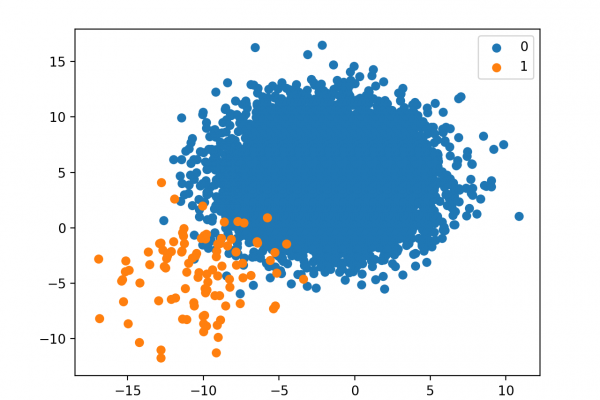# Failure of Classification Accuracy for Imbalanced Class DistributionsLast Updated on January 14, 2020

Classification accuracy is a metric that summarizes the performance of a classification model as the number of correct predictions divided by the total number of predictions.

It is easy to calculate and intuitive to understand, making it the most common metric used for evaluating classifier models. This intuition breaks down when the distribution of examples to classes is severely skewed.

Intuitions developed by practitioners on balanced datasets, such as 99 percent representing a skillful model, can be incorrect and dangerously misleading on imbalanced classification predictive modeling problems.

In this tutorial, you will discover the failure of classification accuracy for imbalanced classification problems.

After completing this tutorial, you will know:

• Accuracy and error rate are the de facto standard metrics for summarizing the performance of classification models.
• Classification accuracy fails on classification problems with a skewed class distribution because of the intuitions developed by practitioners on datasets with an equal class distribution.
• Intuition for the failure of accuracy for skewed class distributions with a worked example.

Kick-start your project with my new book Imbalanced Classification with Python, including step-by-step tutorials and the Python source code files for all examples.

Let’s get started.